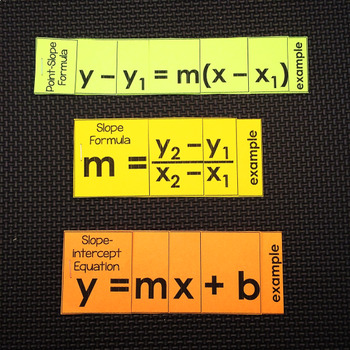# Linear Equations Foldables - print and digital

Rated 4.86 out of 5, based on 532 reviews
532 Ratings;
8th - 9th
Subjects
Resource Type
Standards
Formats Included
• PDF
•Google Apps™
Report this resource to TPTThe Teacher-Author indicated this resource includes assets from Google Workspace (e.g. docs, slides, etc.).
##### Also included in
1. This bundle includes 24 activities for teaching, practicing and reviewing slope and algebraic functions. Also included is a set of Linear Equations Flippables for the slope, slope-intercept and point-slope equations. Many of the activities in this bundle have been updated with digital versions. Beca
Price \$54.00Original Price \$88.50Save \$34.50
2. This bundle contains all of the resources you will need to teach a summer school algebra class. Resources inside cover: solving equations, slope, linear equations, functions &amp; domain and range, polynomials, systems of equations, factoring quadratics and the quadratic formula. For a bundle of eve
Price \$36.00Original Price \$54.50Save \$18.50

### Description

This is a set of 3 flip books for the slope, point-slope and slope-intercept formulas. Each comes in both PDF printable form and digital form in Google Slides.

Two versions of the printable set of flippables are included-- one version has printed explanations of each variable on each flap. The second version is blank other than the variables. This version would work well if you'd like students to take their own notes. At the end of each flippable is an example of the formula in action.

The printables are easily assembled with scissors and a stapler. Each flippable prints on one sheet of paper. These little flip books are perfect in a student notebook or as standalone references throughout the school year. The digital flippables were built in Google Slides.

These foldables are included in: Algebra 1 Activities Bundle w/ digital updates

You may also like:

Graphing Linear Equations Digital Math Escape Room

Slope Digital Math Escape Room

Linear Equations Word Problems Digital Math Escape Room

Algebra Word Wall - print and digital

Total Pages
Included
Teaching Duration
N/A
Report this resource to TPT
Reported resources will be reviewed by our team. Report this resource to let us know if this resource violates TPT’s content guidelines.

### Standards

to see state-specific standards (only available in the US).
Use similar triangles to explain why the slope m is the same between any two distinct points on a non-vertical line in the coordinate plane; derive the equation 𝘺 = 𝘮𝘹 for a line through the origin and the equation 𝘺 = 𝘮𝘹 + 𝘣 for a line intercepting the vertical axis at 𝘣.
Interpret the equation 𝘺 = 𝘮𝘹 + 𝘣 as defining a linear function, whose graph is a straight line; give examples of functions that are not linear. For example, the function 𝘈 = 𝑠² giving the area of a square as a function of its side length is not linear because its graph contains the points (1,1), (2,4) and (3,9), which are not on a straight line.
Calculate and interpret the average rate of change of a function (presented symbolically or as a table) over a specified interval. Estimate the rate of change from a graph.